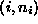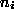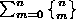Data Structures and Algorithms with Object-Oriented Design Patterns in C## Projects

1. Complete the SetAsArray class introduced in Programby providing suitable definitions for the following operations: Purge, IsEmpty, IsFull, Count, Accept, and GetEnumerator. Write a test program and test your implementation.
2. Complete the SetAsBitVector class introduced in Programby providing suitable definitions for the following methods: Purge, IsEmpty, IsFull, Count, Accept, and GetEnumerator. Write a test program and test your implementation.
3. Rewrite the Insert, Withdraw, and IsMember methods of the SetAsBitVector implementation so that they use bitwise shift and mask operations rather than division and modulo operations. Compare the running times of the modified methods with the original ones and explain your observations.
4. Complete the MultisetAsArray class introduced in Programby providing suitable definitions for the following methods: Purge, Count, Accept, and GetEnumerator. Write a test program and test your implementation.
5. Complete the MultisetAsLinkedList class introduced in Programby providing suitable definitions for the following methods: Purge, IsEmpty, IsFull, Count, CompareTo, Accept, and GetEnumerator. Write a test program and test your implementation.
6. Design and implement a multiset class in which the contents of the set are represented by a linked list of ordered pairs of the form, where i an the element of the universal set U andis a non-negative integer that counts the number of instances of the element i in the multiset. (See Exercisesand).
7. Write a program to compute the number of ways in which a set of n elements can be partitioned. That is, computewhereHint: See Section.Copyright © 2001 by Bruno R. Preiss, P.Eng. All rights reserved.